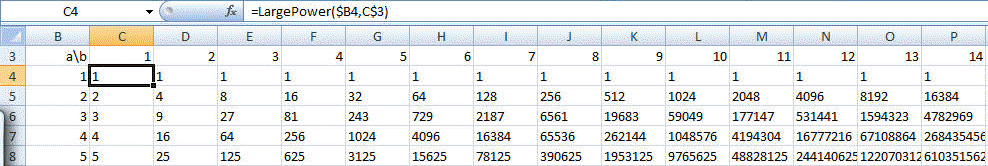﻿ Project Euler Problem 56
You are on the Home/Other Tutorials/Project Euler/Problem 56 pageShare Your

# Project Euler - Problem 56

## Problem description

A googol (10100) is a massive number: one followed by one-hundred zeros; 100100is almost unimaginably large: one followed by two-hundred zeros. Despite their size, the sum of the digits in each number is only 1.

Considering natural numbers of the form, ab, where a, b < 100, what is the maximum digital sum?

## Solution

This is easily solved in Excel with the help of the Large Number Arithmetic module, specifically, the LargePower routine.

In some column and some row, enter the numbers 1 through 100.  My choice of rows and columns are shown below.  Note that one did not have to start with 1.  It is almost certain the answer will involve large values of a and b.  So, one could start at 90 or 75 or some such number.

In the cell corresponding to 1^1 enter the formula =LargePower(\$B4,C\$3).  Note the use of mixed (absolute-relative) references.  Copy this formula across to cover all the columns with the numbers 2, 3, ..., 100.  Copy this row's formulas to the rows below that have numbers in the leftmost column.Now, in an identically sized range (I started with row 105, enter the formula =SUM(--MID(C4,ROW(INDIRECT("1:"&LEN(C4))),1)) where C4 refers to the cell above that contains the 1^1 value.  Copy this formula across the columns and down the rows.  In my case this covers the range C106:CX205.

Finally, in some cell enter the formula =MAX(C106:CX205).  This is the desired result.